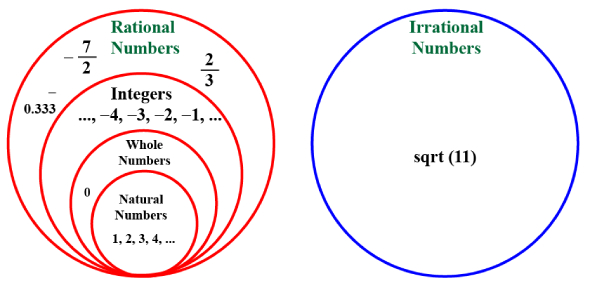# Rational Numbers & Irrational Numbers Quiz

Approved & Edited by ProProfs Editorial Team
At ProProfs Quizzes, our dedicated in-house team of experts takes pride in their work. With a sharp eye for detail, they meticulously review each quiz. This ensures that every quiz, taken by over 100 million users, meets our standards of accuracy, clarity, and engagement.
| Written by Catherinehalcomb
C
Catherinehalcomb
Community Contributor
Quizzes Created: 1509 | Total Attempts: 5,225,587
Questions: 10 | Attempts: 813SettingsHow do you know if a number is rational or irrational? Is 4/5 rational or irrational? Check out our online quiz and attempt some amazing questions to unleash the maths expert.

• 1.

### The decimal expansion of an irrational number may be

• A.

Terminating

• B.

Recurring

• C.

Either terminating or non- terminating

• D.

Non-terminating and non-recurring

D. Non-terminating and non-recurring
Explanation
An irrational number is a number that cannot be expressed as a fraction of two integers. The decimal expansion of an irrational number is non-terminating, meaning it goes on forever without repeating a pattern. Additionally, it is non-recurring, meaning there is no sequence of digits that repeats indefinitely. Therefore, the decimal expansion of an irrational number is both non-terminating and non-recurring.

Rate this question:

• 2.

### The product of a rational and an irrational numbers is:

• A.

Always an integer

• B.

Always an irrational number

• C.

Always a rational number

• D.

Sometimes rational and sometimes irrational

B. Always an irrational number
Explanation
When a rational number (a number that can be expressed as a fraction) is multiplied by an irrational number (a number that cannot be expressed as a fraction), the result is always an irrational number. This is because multiplying a rational number by an irrational number introduces new irrational components that cannot be simplified or expressed as a fraction. Therefore, the product of a rational and an irrational number is always an irrational number.

Rate this question:

• 3.

### The number  is

• A.

An irrational number

• B.

A rational number

• C.

not a natural number

• D.

None of these

B. A rational number
Explanation
A rational number is a number that can be expressed as a fraction, where the numerator and denominator are both integers. Since the question does not provide any specific number, it is not possible to determine if it is a rational number or not. Therefore, the answer "none of these" is the most appropriate.

Rate this question:

• 4.

### For rationalising the denominator of the expression  we multiply and divide by

• A.

• B.

12

• C.

• D.
D.
Explanation
To rationalize the denominator of an expression, we multiply both the numerator and denominator by the conjugate of the denominator. In this case, the conjugate of the denominator is 12. By multiplying and dividing by 12, we can eliminate any irrational terms in the denominator and simplify the expression.

Rate this question:

• 5.

• A.

12

• B.
• C.
• D.
D.
• 6.

### 0.6666  in p/q form is

• A.

6/99

• B.

2/3

• C.

3/5

• D.

1/66

B. 2/3
Explanation
The decimal number 0.6666 can be expressed as a fraction in p/q form as 2/3.

Rate this question:

• 7.

### Which of the following is irrational?

• A.

0.14

• B.

0.24014001400014…

• C.

0.14161416......

• D.

0.1416

B. 0.24014001400014…
Explanation
The number 0.24014001400014… is irrational because it is a non-repeating and non-terminating decimal. Irrational numbers cannot be expressed as a fraction or a ratio of two integers, and they have an infinite number of non-repeating decimal places. Therefore, the number 0.24014001400014… cannot be expressed as a fraction and is considered irrational.

Rate this question:

• 8.

### The square root of which number is rational

• A.

7

• B.

1.01

• C.

0.04

• D.

13

C. 0.04
Explanation
The square root of 0.04 is rational because it can be expressed as 0.2, which is a rational number.

Rate this question:

• 9.

### (16)3/4 is equal to

• A.

2

• B.

4

• C.

8

• D.

16

C. 8
Explanation
The fraction 3/4 can be simplified by dividing the numerator (3) by the denominator (4). The result is 0.75, which can also be expressed as a whole number by multiplying it by 100. Therefore, 0.75 x 100 = 75. Since 75 is divisible by 8, the answer is 8.

Rate this question:

• 10.

### A rational number between  is

• A.

1/14

• B.

2/21

• C.

5/14

• D.

5/21Back to top